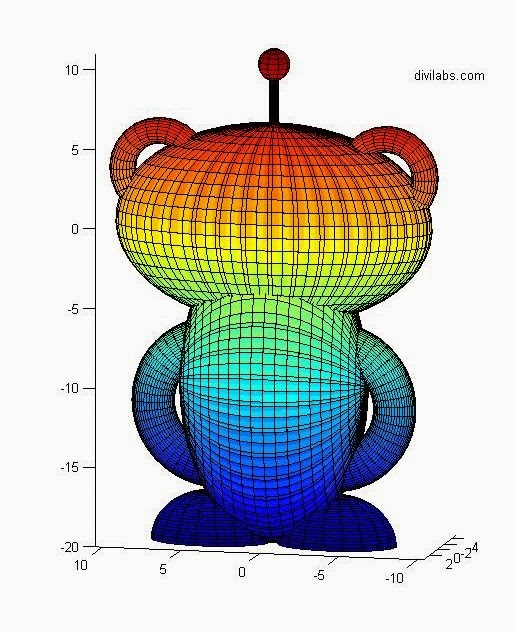# Plotting Reddit dot com Mascot (The Reddit Alien) in MATLAB

The idea of plotting Reddit Mascot (The Reddit Alien), had stricken many people before, who were there in reddit & were a member of subreddit, /r/matlab. But it was Nov. 20, 2013 a reddit user, with the profile link http://www.reddit.com/user/Pilotics has posted this image. And this was the MATLAB plot of reddit mascot, the Reddit Alien.MATLAB Plot of reddit Alien (Reddit MASCOT)
Many have requested him the code of the above plot, but no reply came. But on April 20, 2014, he replied with the code. And the code is:

hold on
view([20 10])

X=linspace(-2,2*pi,50); Y=linspace(0,2*pi,50);
[Y X]=meshgrid(Y,X);
x=-5.*cos(X).*-2.*sin(Y);
y=-2.*sin(Y).*-2.*sin(X);
z=-sqrt(40).*cos(Y);
surf(x,y,z)

% Antenna
clc % Stalk
R = .25;
Rs = linspace(0, R, 4);
SideH = 5;
Sides = 0:SideH;
Azs = 0:15:360;
[AzMesh1, ZMesh] = meshgrid(Azs, Sides);
XMesh1 = (R * cosd(AzMesh1));
YMesh1 = (R * sind(AzMesh1));
surf(XMesh1, YMesh1, ZMesh+5);
clc % End
R = 1; N = 10;
Ang1 = linspace(0,360 ,N); Ang2 = linspace(-90,90,N);
[Ang1s, Ang2s] = meshgrid(Ang1,Ang2);
X = ([cosd(Ang2s) .* cosd(Ang1s)])*R;
Y = ([cosd(Ang2s) .* sind(Ang1s)])*R;
Z = ([sind(Ang2s)]+10)*R;
surf(X, Y, Z);

% Eyes
clc
for A = -2:4:2
R = 1.75; N = 25;
Ang1 = linspace(0,360 ,N); Ang2 = linspace(-90,90,N);
[Ang1s, Ang2s] = meshgrid(Ang1,Ang2);
X = ([cosd(Ang2s) .* cosd(Ang1s)]-A)*R;
Y = ([cosd(Ang2s) .* sind(Ang1s)]-1.75)*R;
Z = ([sind(Ang2s)]+.75)*R;
surf(X, Y, Z);
end

% Smile
clc
Space = linspace(-pi,0,50);
[Thetas, Phis] = meshgrid(Space, Space);
Xarr = (cos(Thetas) .* (7 + cos(Phis)))/1.75;
Yarr = (sin(Phis))-3;
Zarr = ((sin(Thetas) .* (1.85 + cos(Phis)))-1);
surf(Xarr, Yarr, Zarr);

% Ears
clc
for A = -9:18:9
Space = linspace(0,2*pi,25);
[Thetas, Phis] = meshgrid(Space, Space);
Xarr = ((cos(Thetas) .* (3 + cos(Phis)))+A)/1.25;
Yarr = (sin(Phis));
Zarr = ((sin(Thetas) .* (3 + cos(Phis)))+4)/1.25;
surf(Xarr, Yarr, Zarr);
end

% Body
clc
X=linspace(-2,2*pi,50); Y=linspace(0,2*pi,50);
[Y X]=meshgrid(Y,X);
z=(-5.*cos(X).*-2.*sin(Y))-10;
y=(-2.*sin(Y).*-2.*sin(X));
x=(-sqrt(35).*cos(Y));
surf(x,y,z)

% Arms
clc
for A = -2.75:5.5:2.75
Space = linspace(0,2*pi,50);
[Thetas, Phis] = meshgrid(Space, Space);
Xarr = ((cos(Thetas) .* (3 + cos(Phis)))+A)/.75;
Yarr = (sin(Phis));
Zarr = ((sin(Thetas) .* (3 + cos(Phis)))-8.5)/.75;
surf(Xarr, Yarr, Zarr);
end

% Feet
clc
for A = -4:8:4
X=linspace(-2,2*pi,25); Y=linspace(pi,pi/2,25);
[Y X]=meshgrid(Y,X);
x=(-5/2.*cos(X).*-3/2.*sin(Y))-A;
y=(-3/2.*sin(Y).*-3/2.*sin(X));
z=(-sqrt(10).*cos(Y))-20;
surf(x,y,z)
end

text(-8, -4, 9, 'divilabs.com');

axis equal
hold off

The code looks, very complicated, as it deals with the geometry & the plane orientation. But its working fine. So here is the result of our simulation of the above MATLAB code to plot the reddit alien.Plotting Reddit dot com Mascot (The Reddit Alien) in MATLAB Front ViewPlotting Reddit dot com Mascot (The Reddit Alien) in MATLAB Back View
So just copy the code above, & let your imagination work to get various changes to it.

Special thanks & congrats to the user @Politics for this brilliant piece of work.